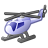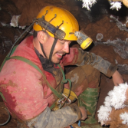# Calculate distance from IRing behaves inconsistant to IProximityOperator

400
2
02-21-2014 01:56 AMOccasional Contributor
Hey around,

I have a two featureClasses of type Polygon where the slave-class depends on the master-class. Having said this I want to reshape some portions of the slave-class (I call them Candidates within my code) by the appropriate paths within the master-class.

So I start up querying the slaves that belong to a given master-features by using a spatial query (intersect). Now I loop every point within the slave and compare them to the points within the master.
I assume the following condition for my candidate:
The from-point and the to-point of the Candidate coincide the master-geometry.
So I calculate the distance of the points within the slave-geometry to the master-geometry using the IProximityOperator-interface (with master-geom as proxy). A distance of 0 indicates that the point is on the master-geometry. So I build up the candidates as ICurve-members by adding all the points that do NOT coincide the master-geometry and finally append the from- and to-point (which DO coincide). Now I have a set of candidates that represent ICurve-members.

The following code retrieves the candidates:

```IPointCollection4 points = (IPointCollection4)slave.Feature.Shape; // the points of the slave-feature

IPoint fromPoint = null;     // fromPoint of the candidate
IPoint toPoint = null;     // toPoint of the candidate

IPolyline curve = new PolylineClass();   // the geometry of the candidate to add to the result-list
IPointCollection curvePoints = (IPointCollection)curve;

for (int j = 19; j < 22; j++)    // consider only these three points
{
// iterate through the points of the slave-geometry

IPoint point = points.get_Point(j);
double distance = proxy.ReturnDistance(point); // distance from every point within the slave to the master-geometry

if (distance > TestClass.Tolerance) // point is totally outside
{
// does not happen in our test-case
// ...
}
else if (distance < TestClass.Tolerance && distance > 0) // point is within the tolerance so it might be a candidate
{
curvePoints.AddPoint(point);
}
else
{

// point touches the outline of the feature. These touching points form the borders of the correction

if (fromPoint == null) fromPoint = point; // set the fromPoint if not exists
toPoint = point;    // set the toPoint

// terminate the candidate and append it to the result

IRelationalOperator relOp = (IRelationalOperator)fromPoint;
if (!relOp.Equals(toPoint))
{
// terminate the current curve

// add the fromPoint to the curve
object before = (object)0;
object after = Type.Missing;
curvePoints.AddPoint(fromPoint, ref before, ref after);
// add the toPoint to the curve
curvePoints.AddPoint(toPoint);

fromPoint = point;  // make the current toPoint the next fromPoint
toPoint = null;

Candidate candidate = new Candidate(curve);// build a new (temporary) candidate from the curve
result.Add(candidate);
}
}

}
```

What I do next is getting the actual reshaper-geometry from the master-feature. Therefor I extract the portion of the master-geometry that fits from- and to-point of my candidate using this code:
```IPoint fromPoint = this.Geometry.FromPoint; // "this" is the candidate we retrieved in the previos code
IPoint toPoint = this.Geometry.ToPoint;
double fromDistance = 0, toDistance = 0;   // distances along the curve
// if these variables are not equal 0 the given point does not belong to the curve
double fromAcrossDistance = 0, toAcrossDistance = 0; // distances across the curve

// get the ring that forms the masterGeometry in order to access the methods for querying near points
IRing ring = new RingClass();
((ISegmentCollection) ring).AddSegmentCollection((ISegmentCollection) masterGeometry);

// calculate the distance of the fromPoint to the master-geometries along its curve
ring.QueryPointAndDistance(esriSegmentExtension.esriNoExtension, fromPoint, false, null, ref fromDistance, ref fromAcrossDistance, false);
// calculate the distance of the toPoint to the master-geometries along its curve
ring.QueryPointAndDistance(esriSegmentExtension.esriNoExtension, toPoint, false, null, ref toDistance, ref toAcrossDistance, false);
// when either the from- or the toPoint does not coincide with the geometry we return an empty curve

// obviously here is the problem
// although we got the from- and toPoints of the candidate (this) by checking if their distance to the masterGeometry is 0 we get some (quite small)
// difference to 0 in the distances accross the curve
if (fromAcrossDistance > 0 || toAcrossDistance > 0) return (ICurve) new Path();
```

What is strange in here is that for the same candidate (whose from- and to-point are on the master-geometry, compare condition) we get from/toAcrossDistances that are not exactly 0 but sth. around 0.0000009 or so. why do IRing#QueryPointAndDistance and IProximityOperator#ReturnDistance behave so different and how can I avoid this?

I hope that you can follow my thoughts and help me finding out the issue behind. I append two shape-files with the mentioned features also.

Bye
Tags (5)
2 RepliesbyMVP Frequent Contributor
This sounds like a precision issue. Although you have supplied a sample of your data as Shapefiles is this in fact the storage format you are using? Are you actually using a geodatabase and the XY resolution of the datasets are different? If they are Shapefiles try importing them into a geodatabase with a default XY resolution, then try your code?

DuncanOccasional Contributor
The featureClasses are indeed in different datasets, but their tolerance is the same (predefined in our database-schema). Actually this tolerance is much greater then the obtained error, while the former is set to 0.0001m (for the whole SDE) the latter is around 0.0000000009 as mentioned before, so I wonder why the engine recognizes this difference anyway...
Both FCs are stored within an SDE.